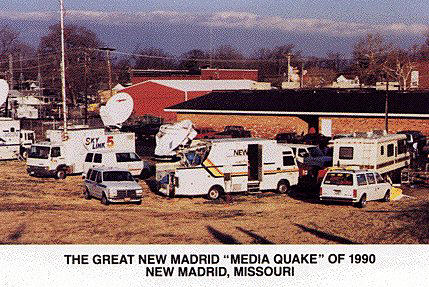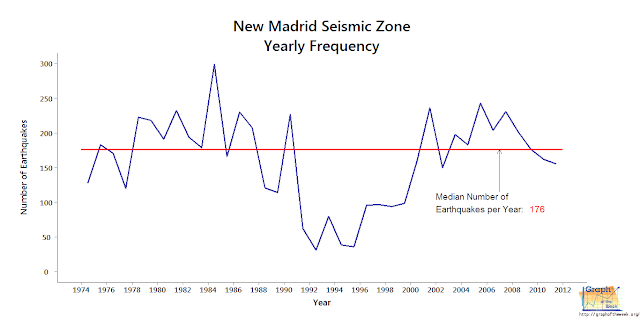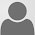## Friday, November 2, 2012

### The New Madrid Fault - Past, Present and Future

New Madrid, Territory of Missouri, March 22, 1816
Dear Sir,
In compliance with your request, I will now give you a history, as full in detail as the limits of the letter will permit, of the late awful visitation of Providence in this place and vicinity.
On the 16th of December, 1811, about two o'clock, A.M., we were visited by a violent shock of an earthquake, accompanied by a very awful noise resembling loud but distant thunder, but more hoarse and vibrating, which was followed in a few minutes by the complete saturation of the atmosphere, with sulphurious vapor, causing total darkness. The screams of the affrighted inhabitants running to and fro, not knowing where to go, or what to do - the cries of the fowls and beasts of every species - the cracking of trees falling, and the roaring of the Mississippi - the current of which was retrograde for a few minutes, owing as is supposed, to an irruption in its bed -- formed a scene truly horrible.
[Remaining text of this letter not shown]
Eliza BryanCourtesy of Wikipedia
It is hard to truly imagine the disaster described by Miss Bryan*. Sadly, there were no camcorders at the time so there isn't any first-hand video revealing the pandemonium. However, the depiction above shows us something of the experience.

*Fantastic reading about Bryan's and other first-hand accounts can be found in Norma Bagnall's On Shaky Ground: The New Madrid Earthquakes of 1811-1812.

Before this particular cataclysm, the New Madrid Seismic Zone had been a real tempest a number of times. Similar in magnitude and destruction as seen above, it rocked the area in A.D. 1450 and A.D. 900. As noted by Tuttle, et. al. in their publication "The Earthquake Potential of the New Madrid Seismic Zone" (link opens PDF file):

Liquefaction data indicate that New Madrid events occurred every 500 ( +/- 300) years during the past 1200 years.

Well, let's see where things stand, on this bicentennial anniversary of the last 'big one.'

New Madrid Seismic Zone, Present Day (2012)

Ever been to Reelfoot Lake?

If you have, then you can thank the historic earthquake mentioned above and its brethren for creating it. During the winter of 1811-1812, four - count 'em - four - magnitude 7 or greater quakes hit the New Madrid area (there were many smaller aftershocks during the period). In case you're wondering where that is, it's a large region where the states of Tennessee, Arkansas, Missouri, Illinois and Kentucky all meet:Courtesy of USGS - link opens PDF file

For two hundred years, this area hasn't felt any quakes that compare to the whoppers mentioned above. Only two have registered higher than 5.0: a 6.6 quake in 1895, and a 5.4 temblor in 1968.

Breaking News: Memphis felt an earthquake on Monday, October 29, 2012 at 7:39 a.m. Being magnitude 3.9, it went almost entirely unnoticed. The epicenter was located in Parkin, AR (about an hour's drive west from Memphis).

In 1974, monitoring equipment was installed in the area to track geological activity, allowing us to chart the resulting movement:Figure 1 (click on graph to enlarge)

When we examine this graph, a number of items become clear:
1. The 1811-12 earthquakes were big. A sparsely-populated region prevented this quake from having more notoriety, otherwise it would rank up there with the San Francisco quake of 1906.
2. The yearly median quake size in the New Madrid region is extremely low, hovering around magnitude 1.6. Even though there have been over 4000 quakes since 1974, only 2 have been large enough to be felt - the rest are tiny.
3. The largest measured annual earthquake in the New Madrid region has never risen past magnitude 5.0 since 1974.
4. There is a trend since 2001 indicating that the system is in equilibrium.

So what does all of that mean? Will there be another huge earthquake or not?

New Madrid Fault - the Future

We haven't seen any major quakes coming out of the New Madrid fault, despite predictions as well as stories about the next 'big one.' Perhaps the best remembered prognosticator was one Iben Browning who created a local uproar when he predicted a major earthquake for the area which was to occur on December 2 or 3, 1990. The media frenzy which descended upon New Madrid during that time was unlike anything that town had probably ever seen:Courtesy of Show-Me
Fact: the author of this article lived in Memphis at the time of Browning's prediction and insisted that his landlord include insurance protection against earthquakes in the rental agreement. Who's to say I can't get worked up with the best of 'em?

Is It Shutting Down?

There is compelling evidence that the New Madrid fault is in fact, shutting down. Seth Stein, a geology professor at Northwestern, championed this notion in Disaster Deferred: A New View of Earthquake Hazards in the New Madrid Seismic Zone. Using GPS data to measure land movement in the area, he discovered that it's barely moving:

"Our GPS data for New Madrid didn't show any motion. Specifically, they showed that the ground was moving less than 2 millimeters per year. That's at least 18 times more slowly than the San Andreas. We were also pretty sure that the number would get much smaller if we kept measuring for a longer time. Already it was a lot slower than we'd expect if a big earthquake were coming any time soon."

Further, he goes on to suggest that predicting earthquakes in the area is problematic at best:

"Geologists know surprisingly little about what's going on here. We don't know why earthquakes occur; when they started; if, when, and where future large earthquakes will occur; how serious a danger they pose; or how society should confront them."

Looking back at the first graph once again (Figure 1), the last thirty-eight years show the New Madrid fault displaying remarkable stability. However, that's a mere sliver of time in the grand history of this area, so cannot be relied upon as a predictor of future events.

Perhaps looking at the number of quakes each year will yield something interesting:Figure 2 (click on graph to enlarge)
176 tremors per year (median) means that some sort of shock hits this region every two days or so. That seems like a lot until compared with the San Andreas fault, which checks in at seventy tremors per day! If anything, however, this would support the argument that the New Madrid fault is shutting down (if only we had these measurements dating back a thousand years...).

It is rather interesting to note that for a few years in the 1990s the level of activity was extraordinarily low - even for this area. Ironically, this is the period during which Browning predicted a big quake would occur!

Or Is It Cranking Up?

The United States Geological Survey (USGS), as recently as 2008, will tell you that a large earthquake can arrive anytime (link opens PDF file):

The panel concludes that the New Madrid Seismic zone is at significant risk for damaging earthquakes that must be accounted for in urban planning and development.

That's not much of a prediction and in fact supports Stein's statement that geologists know 'surprisingly little' about what might occur. In fact, if you asked just about anybody around these parts, they would say the same thing (albeit in more plain language!).

Mainstream geologists will agree with the USGS. "The Earthquake Potential of the New Madrid Seismic Zone" (link opens PDF file) states:

On the basis of a minimum recurrence rate of 200 years, we are now entering the period during which the next 1811–1812-type event could occur.

And how big is that next period where an '1811-1812-type' event could occur? 800 - that's eight hundred - years. To be fair, time frames associated with faults span thousands of years (if not more), so it's difficult to achieve better precision.

Conclusion

So what's the verdict? Is it shutting down or cranking up? The author hoped to find strong evidence one way or the other, but alas, it just isn't to be found - yet. Given the amount of data out there on the topic, one would think we knew everything about these things. In other words, your guess is as good as mine.

However, perhaps there is a correlation here that everybody missed - except for Eliza Bryan. Here is the post script to her letter mentioned above:

There is one circumstance which I think worthy of remark. This country was formerly subject to very hard thunder; but for more than twelve months before the commencement of the earthquake there was none at all, and but very little since, a great part of which resembles subterraneous thunder. The shocks still continue, but are growing more light, and less frequent. -E.B.

Thunder? A predictor of earthquakes? I like it. Next time things get a little too quiet around your neighborhood, better be prepared for the next 'big one.'

P.S. While the author does not believe any large earthquake is coming to the New Madrid area anytime soon (meaning within my lifetime), your opinion may differ. Feel free to purchase a survival kit in case of such an emergency.

Questions:
1) Do other fault systems exhibit similar traits as the New Madrid system?
2) What is your prediction on whether or not another devastating earthquake will occur in this region?
3) What is the best way to predict earthquakes?

Data:
Related Information:

Code:
These graphs were generated using the 'ggplot2' and 'maps' packages within the R programming language. Additional graphics were created/edited using GIMP.
```
1st graph:
ggplot() +
geom_rect(aes(xmin=1972, xmax=2012, ymin=0, ymax=2.49), fill="gray", alpha=0.1) +
geom_rect(aes(xmin=1972, xmax=2012, ymin=2.5, ymax=3.99), fill="gray", alpha=0.25) +
geom_rect(aes(xmin=1972, xmax=2012, ymin=4, ymax=5.99), fill="gray", alpha=0.5) +
geom_rect(aes(xmin=1972, xmax=2012, ymin=6, ymax=7.99), fill="gray", alpha=0.75) +
geom_rect(aes(xmin=1972, xmax=2012, ymin=8, ymax=10), fill="gray", alpha=1.0) +

geom_text(aes(x=1972, y=2.5), label="Not felt", size=3, color="black", alpha=0.6, hjust=0, vjust=1) +
geom_text(aes(x=1972, y=4), label="Minor", size=3, color="black", alpha=0.6, hjust=0, vjust=1) +
geom_text(aes(x=1972, y=6), label="Damaging", size=3, color="black", alpha=0.6, hjust=0, vjust=1) +
geom_text(aes(x=1972, y=8), label="Devastating", size=3, color="black", alpha=0.6, hjust=0, vjust=1) +
geom_text(aes(x=1972, y=10), label="Hell on Earth", size=3, color="black", alpha=0.6, hjust=0, vjust=1) +

geom_segment(aes(x=1973, xend=1973, y=9.7, yend=8), size=0.2, color="black", alpha=0.6, linetype=2, arrow = arrow(length = unit(0.3, "cm"))) +
geom_segment(aes(x=1973, xend=1973, y=7.7, yend=6), size=0.2, color="black", alpha=0.6, linetype=2, arrow = arrow(length = unit(0.3, "cm"))) +
geom_segment(aes(x=1973, xend=1973, y=5.7, yend=4), size=0.2, color="black", alpha=0.6, linetype=2, arrow = arrow(length = unit(0.3, "cm"))) +
geom_segment(aes(x=1973, xend=1973, y=3.7, yend=2.5), size=0.2, color="black", alpha=0.6, linetype=2, arrow = arrow(length = unit(0.3, "cm"))) +
geom_segment(aes(x=1973, xend=1973, y=2.3, yend=0), size=0.2, color="black", alpha=0.6, linetype=2, arrow = arrow(length = unit(0.3, "cm"))) +

geom_segment(aes(x=1972, xend=2012, y=8.1, yend=8.1), size=1.1, color="red", alpha=0.8) +

geom_text(aes(x=1993, y=8.5), label="1811 New Madrid Earthquake (8.1)", size=6, color="red", alpha=0.8) +

geom_point(data=yearly.max, aes(x=Year, y=Max,color="Largest Annual Earthquake"), size=2, alpha=0.7) +
geom_line(data=yearly.max, aes(x=Year, y=Max, color="Largest Annual Earthquake"), size=1.2, alpha=0.5) +

geom_point(data=yearly.median, aes(x=Year, y=Median, color="Median Annual Earthquake"), size=2, alpha=0.7) +
geom_line(data=yearly.median, aes(x=Year, y=Median, color="Median Annual Earthquake"), size=1.2, alpha=0.5) +

ylab("Magnitude\n") +
xlab("Year") +

scale_y_continuous(limits=c(0, 10), breaks=seq(0,10,by=1)) +
scale_x_continuous(limits=c(1972, 2012), breaks=seq(1974, 2012, by=2)) +

scale_colour_manual(values=palette) +

opts(
plot.title=theme_text(size=24, family='Segoe'),
title = "New Madrid Seismic Zone\nYearly Activity",
legend.title = theme_blank(),
legend.text = theme_text(size=10, family='Segoe'),
legend.position = "right",
panel.background = theme_blank(),
panel.grid.major = theme_blank(),
panel.grid.minor = theme_blank(),
panel.margin = unit(.1, "cm"),
axis.line = theme_segment(),
axis.text.x=theme_text(size=12, family='Segoe'),
axis.text.y=theme_text(size=12, family='Segoe'),
axis.title.x=theme_text(size=14, vjust=-0.4, family='Segoe'),
axis.title.y=theme_text(size=14, hjust=0.5, angle=90, family='Segoe'),
plot.margin = unit(c(1, 0, 1, 1), "cm"))

2nd graph:
ggplot() +
geom_freqpoly(data=yearly.count, aes(x=Year, weight=UTC), color=alpha("dark blue", 0.7), size=1.1, binwidth=1, na.rm= TRUE) +
geom_segment(aes(x=1974, xend=2012, y=176, yend=176), size=1.1, color="red", alpha=0.8) +

geom_segment(aes(x=2007, xend=2007, y=115, yend=176), size=0.2, color="black", alpha=1, arrow = arrow(length = unit(0.3, "cm"))) +
geom_text(aes(x=2002, y=100), label="Median Number of\nEarthquakes per Year:", size=5, color="black", angle=0, alpha=0.8, hjust=0) +
geom_text(aes(x=2009.4, y=91), label="176", size=5, color="red", angle=0, alpha=1, hjust=0) +

ylab("Number of Earthquakes\n") +
xlab("Year") +

scale_y_continuous(limits=c(0, 300), breaks=seq(0,300,by=50)) +
scale_x_continuous(limits=c(1974, 2012), breaks=seq(1974, 2012, by=2)) +

opts(
plot.title=theme_text(size=24, family='Segoe'),
title = "New Madrid Seismic Zone\nYearly Frequency",
legend.title = theme_blank(),
panel.background = theme_blank(),
panel.grid.major = theme_blank(),
panel.grid.minor = theme_blank(),
axis.line = theme_segment(),
axis.text.x=theme_text(size=12, family='Segoe'),
axis.text.y=theme_text(size=12, family='Segoe'),
axis.title.x=theme_text(size=14, vjust=-0.4, family='Segoe'),
axis.title.y=theme_text(size=14, hjust=0.5, angle=90, family='Segoe'),
plot.margin = unit(c(1, 3, 1, 1), "cm"))

```

1.1.